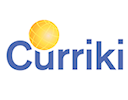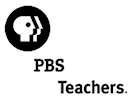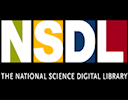Search IntMath
Close

# Free math resources

By Murray Bourne, 22 Feb 2011

Here's a list of free math resources.

The first ones are relevant for students and teachers (and parents!), while the ones at the end are more appropriate for teachers.

## Curriki (a curriculum wiki)For: Students and teachers Curriki is a place where students, parents and teachers can share learning resources. You can easily search the site for math resources. Why not add some yourself?

## FREE (Federal Resources for Educational Excellence)For: Students and teachers This is from government agencies in the US. Go here for the Math Topics. Update: FREE no longer exists!

## NASAFor: Students and teachers Do a search on "math". You will find topics like:

The rest of the resources are aimed at teachers in particular.

## PBSFor: Teachers PBS is one of the best resources in the US. Here are the Math Classroom Resources

## NSDL (National Science Digital Library)For: Teachers This set of resources is aimed at addressing state standards in math

## Teachers' DomainFor: Teachers This is a place where teachers can download, share and save resources. Free registration. Mathematics resources

## TeachnologyFor: Teachers This is another online teacher resource. Math Skills and Topics

This list of resources is based on "Ten great sites with free teacher resources" from e-School News.

### Comment Preview

HTML: You can use simple tags like <b>, <a href="...">, etc.

To enter math, you can can either:

1. Use simple calculator-like input in the following format (surround your math in backticks, or qq on tablet or phone):
a^2 = sqrt(b^2 + c^2)
(See more on ASCIIMath syntax); or
2. Use simple LaTeX in the following format. Surround your math with $$ and $$.
$$\int g dx = \sqrt{\frac{a}{b}}$$
(This is standard simple LaTeX.)

NOTE: You can mix both types of math entry in your comment.